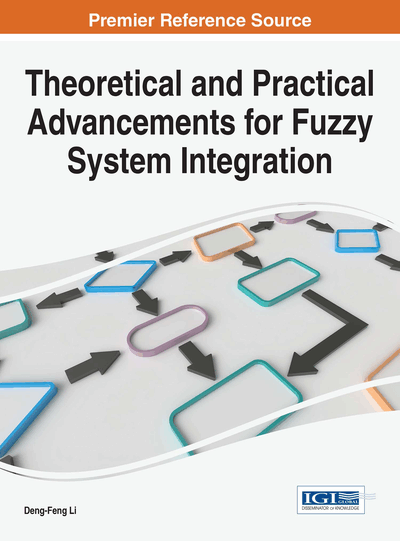# MAGDM Problems with Correlation Coefficient of Triangular Fuzzy IFS

John Robinson P. (Bishop Heber College, India) and Henry Amirtharaj E. C. (Bishop Heber College, India)
DOI: 10.4018/978-1-5225-1848-8.ch007

## Abstract

Correlation coefficient of Intuitionistic Fuzzy Set (IFS), Interval valued IFS, Triangular IFS and Trapezoidal IFS are already present in the literature. This paper proposes the correlation coefficient for Triangular Fuzzy Intuitionistic Fuzzy set (TrFIFS). The method on uncertain Multiple Attribute Group Decision Making (MAGDM) problems based on aggregating intuitionistic fuzzy information is investigated for TrFIFSs. The Triangular Fuzzy Intuitionistic Fuzzy Ordered Weighted Averaging (TrFIFOWA) operator is proposed for TrFIFSs and the Triangular Fuzzy Intuitionistic Fuzzy Ordered Weighted Geometric (TrFIFOWG) operator is utilized for decision making models where expert weights are completely unknown. Based on these operators and the correlation coefficient defined for the TrFIFSs, new decision making models are proposed with numerical illustrations. Some comparisons are also made with existing ranking methods for validity.
Chapter Preview
Top

## Introduction

Decision making is the study of identifying and choosing alternatives based on the values and preferences of the decision maker. Making a decision implies that there are alternative choices to be considered, and in such cases many of these alternatives as possible should be identified and the one that best fits with our goals, objectives, desires, values, should be chosen (Kahraman, 2008). Decision making should start with the identification of the decision maker(s) and stakeholder(s) in the decision, reducing possible disagreement about problem definition, requirements, goals and criteria. It is very important to make a distinction between the cases where we have a single or multiple criteria. When a decision problem has a single criterion or a single aggregate measure, then the decision can be made implicitly by determining the alternative with the best value of the single criterion or aggregate measure. When a decision problem has a finite number of criteria or multiple criteria, and the number of the feasible alternatives (the ones meeting requirements) is infinite, and then the decision problem belongs to the field of multiple criteria optimization. Also, techniques of multiple criteria optimization can be used when there are a finite number of feasible alternatives, but are given only in implicit form. This research work focuses on decision making problems when the number of the criteria (attribute) and alternatives is finite, and the alternatives are explicitly given. Problems of this type are called Multi Attribute Decision Making (MADM) problems.

## Complete Chapter List

Search this Book:
Reset